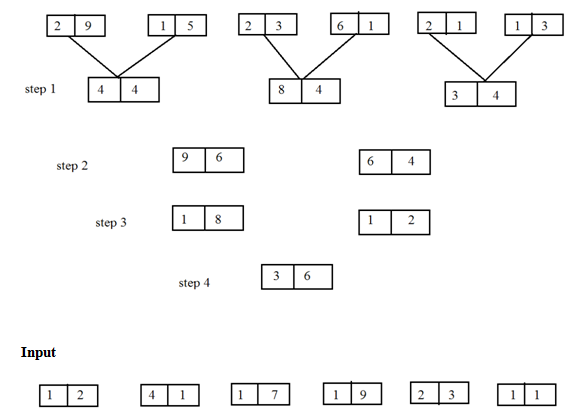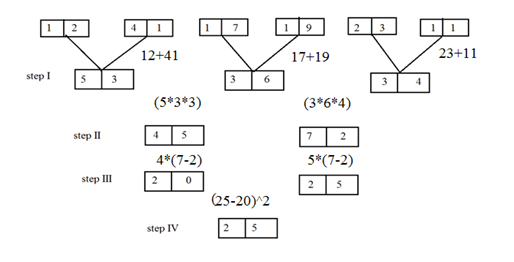# Crack SBI Clerk / IDBI Executive 2018 – Logical Reasoning (Input Output and Inequality) Day-49

Dear Readers, Reasoning Section play a vital role in Banking and all other competitive exams. This year 2018 was started with three wonderful opportunities; Most Awaited SBI Clerk 2018. To enrich your preparation here we have providing new series of Practice Questions on Reasoning Ability – Input Output and Inequality. Candidates those who are going to appear in Bank Exams 2018 can practice these questions daily and make your preparation effective.

[WpProQuiz 1791]

Click “Start Quiz” to attend these Questions and view Explanation1) Find the addition of the three numbers obtained in step 1?

a) 125

b) 124

c) 123

d) 126

e) None of these

2) Find the difference between two numbers obtained in Step III?

a) 6

b) 10

c) 12

d) 5

e) None of these

3) Find the Multiplication of two numbers obtained inStep II?

a) 3248

b) 3240

c) 3180

d) 3255

e) None of these

4) Find the cube of number which is obtained in Step IV??

a) 10648

b) 12167

c) 17576

d) 15625

e) 1000

5) If digit is exchanged within the each block then find the addition of two new numbers obtained in step II?

a) 87

b) 98

c) 81

d) 99

e) None of these

6)Which of the following expressions will be true if the expression O≥ Z> X = Y> S

a) Z < S

b) Z>S

c) S >Y

d) S = O

e) None of these

7)Which of the following symbols should replace the question mark (?) in the given expression in order to make the expressions B>D as well as U≤V definitely true?

B>V? D ≥ N = U

a) ≤

b) >

c) <

d) ≥

e) Either ≤ or <

8)Which of the following symbols should be placed in the blank spaces respectively (in the same order from left to right) in order to complete the given expression in such a manner that makes the expression Y>Z as well as X≤W definitely true?

Y _ W _ O _ Z _ X

a) =, =, ≥, ≥

b) >, ≥, =, >

c) >, <, =, ≤

d) >, =, =, ≥

e) >, =, ≥, >

9)Which of the following should be placed in the blank spaces respectively (in the same order from left to right) in order to complete the given expression in such a manner that makes the expression Z> Xdefinitely true?

_ ≤ _ < _ > _

a) L, N, X, Z

b) L, Z, X, N

c) Z, L, X, N

d) N, Z, X, L

e) X, N, Z, L

10)Which of the following symbols should be placed in the blank spaces respectively (in the same order from left to right) in order to complete the given expression in such a manner that makes the expression A>D and C>E definitely false?

A _ B _ C _ D_ E

a) <, <, >, =

b) <, =, =, >

c) <, =, =, <

d) ≥, =, >, ≥

e)>, >, =, <

Directions (1-5):53+36+34=123

25 – 20 =  5

45*72 = 3240

25*25*25 = 15625

54+27 =81

Direction (6-10)

8. Answer d)>, =, =, ≥

9. Answer  e)X, N, Z, L

10. Answer c) <, =, =, <

Daily Practice Test Schedule | Good Luck

 Topic Daily Publishing Time Daily News Papers & Editorials 8.00 AM Current Affairs Quiz 9.00 AM Logical Reasoning 10.00 AM Quantitative Aptitude “20-20” 11.00 AM Vocabulary (Based on The Hindu) 12.00 PM Static GK Quiz 1.00 PM English Language “20-20” 2.00 PM Banking Awareness Quiz 3.00 PM Reasoning Puzzles & Seating 4.00 PM Daily Current Affairs Updates 5.00 PM Data Interpretation / Application Sums (Topic Wise) 6.00 PM Reasoning Ability “20-20” 7.00 PM English Language (New Pattern Questions) 8.00 PM General / Financial Awareness Quiz 9.00 PM